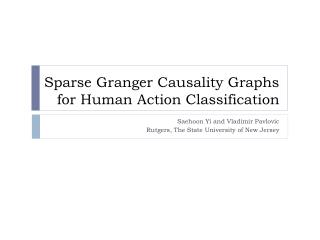DownloadDownload PresentationSparse Granger Causality Graphs for Human Action Classification

Sparse Granger Causality Graphs for Human Action Classification

Télécharger la présentationSparse Granger Causality Graphs for Human Action Classification

- - - - - - - - - - - - - - - - - - - - - - - - - - - E N D - - - - - - - - - - - - - - - - - - - - - - - - - - -
Presentation Transcript

1. Sparse Granger Causality Graphs for Human Action Classification Saehoon Yi and Vladimir Pavlovic Rutgers, The State University of New Jersey

2. Outline • Objective and challenges • Previous work • Sparse Granger Causality Graph Model • Analysis and result • Conclusion

3. Objective • Classify human action time series data Challenges • High dimensional time series data • Dimensionality reduction • Difficulties in interpretation • Idiosyncratic patterns of same action • Need to find commonality within an action

4. Previous work • Learning dynamics of joints • Each action is modeled as Linear Dynamic System • C. Bregler, CVPR 97 • Align time series data • Dynamic Time Warping • Canonical Time Warping • F. Zhou and F. De la Torre, NIPS 2009 • Need to tune parameter for each pair of sequence • Isotonic Canonical Correlation Analysis • S. Shariat and V. Pavlovic, ICCV 2011

5. Our approach • Robust representation of continuous joint movements using micro event point processes. • Models salient and sparse temporal relations among skeletal joints movements

6. Overview

7. Step 1: Generate micro event point processes Continuous time series Joint angles on knees Detect maximal/minimal extreme points as events Micro event point processes

8. Step 2: Estimate Granger Causality Graph • Granger causality in time • Given two AR time series X, Y • Granger causality

9. Granger causality in frequency • Given two point processes , • Estimate power spectrum • Decompose spectrum using Wilson’s algorithm • Granger causality • [A. Nedungadi, G. Rangarajan, N. Jain, and M. Ding ’09]

10. Granger causality graph representation • Estimate Granger causality • for each pair of micro events • f frequencies → summarized to 4 bands

11. Step 3: Learn L1 regularized regression • Input : 16M2 Granger causality features • Output : action category label • Sparse regression coefficient W for each action • Common causality pattern within each class • Positive coefficient Wij • edge i→ j have high causality • Negative coefficient Wij • edge i→ j have low causality

12. Experiments • HDM05 dataset • Motion capture sequence of 29 skeletal joints • Each action is performed by 5 subjects • 8 action classes are chosen

13. Experiment settings • Two different cross validation settings • Cut 1 • Randomly partition training / testing across all subjects • Cut 2 • Test set subjects different from training subjects • To show classification accuracy on unseen data

14. Example of Sparse Granger Causality Graph Model

15. Example of Sparse Granger Causality Graph Model

16. Comparative result Confusion matrix of SGCGM

17. Conclusion • Learn common structure within an action • The sparse regression model chose which pairwise relationship is important for the action • Interpretability of the model • Granger causal graph describes temporal relationship between two joints

18. Thanks you. Q & A